NCERT Class 8 Maths Understanding Quadrilaterals

# NCERT Class 8 Maths Understanding Quadrilaterals

The chapter 3 begins with an introduction to polygons and classification of polygons. Convex and concave polygons, regular and irregular polygons are also described in detail here.The angle sum property which is one of the most useful results is explained in detail here. In the later part of the chapter, different kinds of quadrilaterals such trapezium, kite and parallelograms are described in detail. Then the elements, angles and diagonals of a parallelogram are discussed in the next section of the chapter.Some special parallelograms such as rhombus, rectangle, and square are explained in the last part of the section.

## Chapter 3 Ex.3.1 Question 1

Given here are some figures.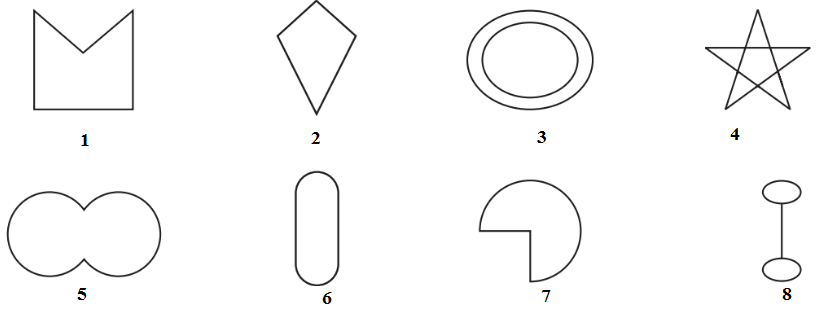Classify each of them on the basis of the following.

(a) Simple curve

(b) Simple closed curve

(c) Polygon

(d) Convex polygon

(e) Concave polygon

### Solution

Steps:

(a) Simple curve - A simple curve is a curve that does not cross itself.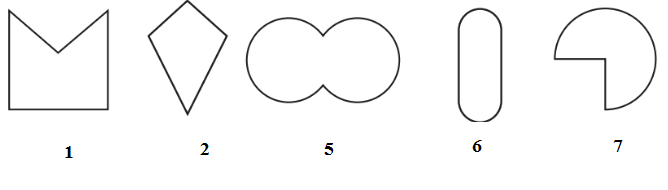(b) Simple closed curve -In simple closed curves the shapes are closed by line-segments or by a curved line.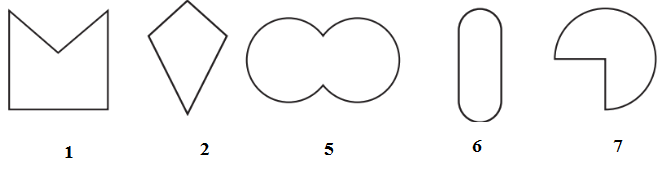(c) Polygon-A simple closed curve made up of only line segments is called a polygon.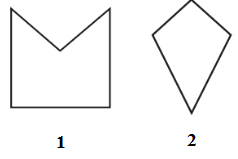(d) Convex polygon-A Convex polygon is defined as a polygon with no portions of their diagonals in their exteriors.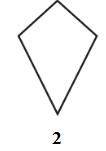(e) Concave polygon- A concave polygon is defined as a polygon with one or more interior angles greater than $$180^{\circ}.$$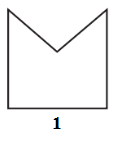## Chapter 3 Ex.3.1 Question 2

How many diagonals does each of the following have?

(b) A regular hexagon

(c) A triangle

### Solution

What is known?

Polygons with known number of vertices.

What is unknown?

Number of diagonals

Reasoning:

A diagonal is a line segment connecting two non-consecutive vertices of a polygon. Draw the above given polygon and mark vertices and then, draw lines joining the two non-consecutive vertices. From this, we can calculate the number of diagonals.

A convex quadrilateral has two diagonals.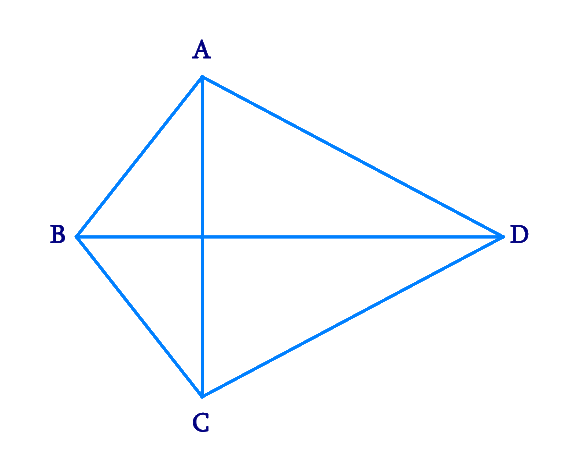Here, $$AC$$ and $$BD$$ are two diagonals.

(b) A regular hexagon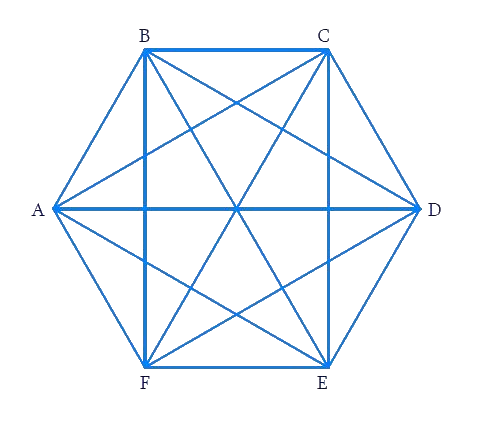Here, the diagonals are $$AD, \,AE,\, BD,\, BE,\, FC,\, FB, \,AC,\, EC$$ and $$FD.$$ Totally there are $$9$$ diagonals.

(c) A triangle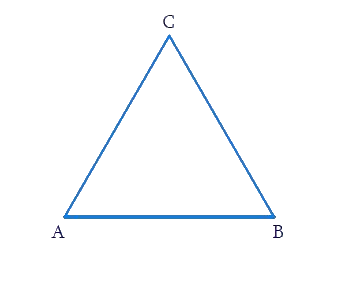A triangle has no diagonal because there no two non-consecutive vertices.

## Chapter 3 Ex.3.1 Question 3

What is the sum of the measures of the angles of a convex quadrilateral? Will this property hold if the quadrilateral is not convex? (Make a non-convex quadrilateral and try!)

What is known?

Quadrilateral $$ABCD$$

What is unknown?

Sum of the measures of the angles of a convex quadrilateral.

Reasoning:

Let $$ABCD$$ be a convex quadrilateral. Then, we draw a diagonal $$AC$$ which divides the Quadrilateral into two triangles. We know that the sum of the angles of a triangle is $$180$$ degree, so by calculating the sum of the angles of a $$∆ABC$$ and , we can measure the sum of angles of convex quadrilateral.

### Solution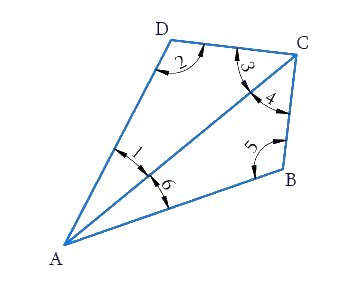$$ABCD$$ is a convex quadrilateral made of two triangles $$∆ABC$$ and $$∆ADC.$$ We know that the sum of the angles of a triangle is $$180$$ degree. So:

\begin{align}\!\angle 6\! +\! \angle 5 \!+ \!\angle 4\! =\! {180^ \circ }\left[ \begin{array}{l}{\rm{Sum}}\,\,{\rm{of}}\,{\rm{the}}\,{\rm{angles}}\,\\{\rm{of}}\,{\rm{\Delta ABC}}\,{\rm{ = 18}}{{\rm{0}}^{\rm{o}}}\end{array} \right]\\ \angle 1\!+ \!\angle2\!+\!\angle 3\!=\!{180^ \circ }\left[ \begin{array}{l}{\rm{Sum}}\,\,{\rm{of}}\,{\rm{the}}\,{\rm{angles}}\,\\{\rm{of}}\,{\rm{\Delta ADC}}\,{\rm{ = 18}}{{\rm{0}}^{\rm{o}}}\end{array} \right] \end{align}

\begin{align}\angle 6 &+ \angle 5 + \angle 4 + \angle 1 + \angle 2 + \angle 3\\ &= {{180}^{\rm{o}}} + {{180}^{\rm{\circ}}}\\ & = {{360}^{\rm{\circ}}}\end{align}

Hence, the sum of measures of the triangles of a convex quadrilateral is $$360^\circ$$. Yes, even if quadrilateral is not convex then, this property applies. Let $$ABCD$$ be a non-convex quadrilateral; join $$BD$$, which also divides the quadrilateral in two triangles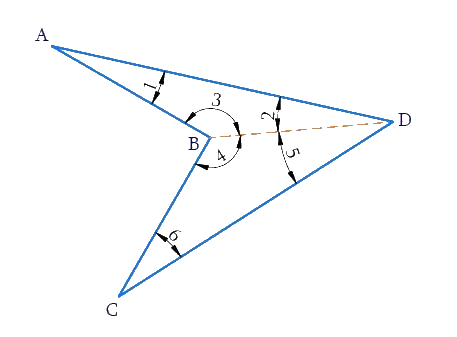Using the angle sum property of triangle, again$$ABCD$$ is a concave quadrilateral, made of two triangles $$\Delta \text{ABD}$$ and $$\Delta \text{BCD}$$ .Therefore, the sum of all the interior angles of this quadrilateral will also be,

$180^\circ + 180^\circ=360^\circ$

## Chapter 3 Ex.3.1 Question 4

Examine the table. (Each figure is divided into triangles and the sum of the angles deduced from that.)

 FIGURES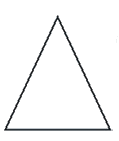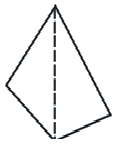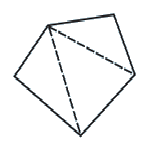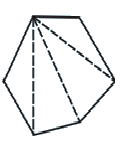SIDE 3 4 5 6 ANGLE SUM $$180$$ \begin{align} 2 \times {180^{\rm{o}}} & = (4 - 2) \times {180^{\rm{o}}}\\ &= 360^\circ \end{align} \begin{align} 3 \times {180^{\rm{o}}}\\ & = (5 - 2) \times {180^{\rm{o}}}\\ & = 540 \end{align} \begin{align} 4 \times {180^{\rm{o}}}\\ & = (6 - 2) \times {180^{\rm{o}}}\\ & = {720^{\rm{o}}} \end{align}

What can you say about the angle sum of a convex polygon with number of sides?

(a) $$7$$

(b) $$8$$

(c) $$10$$

(d) $$n$$

### Solution

From the table, it can be observed that the angle sum of a convex polygon of $$n$$ sides is $$\left( {n - 2} \right){\rm{ }} \times {\rm{ }}180^\circ$$

(a) When $$n = 7$$

Then Angle sum of a polygon

\begin{align} &= (n - 2) \times {180^{\rm{\circ}}} \\&= (7 - 2) \times {180^{\rm{\circ}}} \\&= 5 \times {180^{\rm{\circ}}} \\&= {900^{\rm{\circ}}}\end{align}

(b) When $$n = 8$$

Then Angle sum of a polygon

\begin{align} &= (n - 2) \times {180^{ \rm{ \circ}}} \\&= (8 - 2) \times {180^{\rm{\circ}}} \\&= 6 \times{180^{\rm{\circ}}} \\&= {1080^{\rm{\circ}}}\end{align}

(c) When $$n = 10$$

Then Angle sum of a polygon

\begin{align} &= (n - 2) \times {180^{\rm{\circ}}} \\&= (10 - 2) \times {180^{\rm{\circ}}} \\&= 8 \times {180^{\rm{\circ}}} \\&= {1440^{\rm{\circ}}}\end{align}

(d) When $$n = n$$

Then Angle sum of a polygon $$= \left( {n - {\rm{ }}2} \right) \times 180^\circ$$

## Chapter 3 Ex.3.1 Question 5

What is a regular polygon? State the name of a regular polygon of

(i) $$3$$ sides

(ii) $$4$$ sides

(iii) $$6$$ sides

### Solution

What is known?

Number of sides of polygon

What is unknown?

Name of a regular polygon of

(i) $$3$$ sides

(ii) $$4$$ sides

(iii) $$6$$ sides

Steps:

Regular polygon - A polygon having all sides of equal length and the interior angles of equal measure is known as regular polygon i.e. a regular polygon is both ‘equiangular’ and ‘equilateral

(i) $$3$$ sides $$=$$ polygons having three sides is called a Triangle.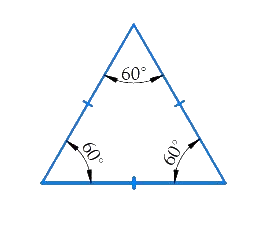(ii) $$4$$ sides $$=$$ polygons having four sides is called square/quadrilateral.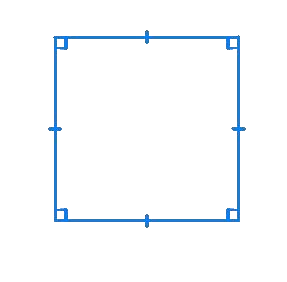(iii) $$6$$ sides $$=$$ polygons having six sides is called a Hexagon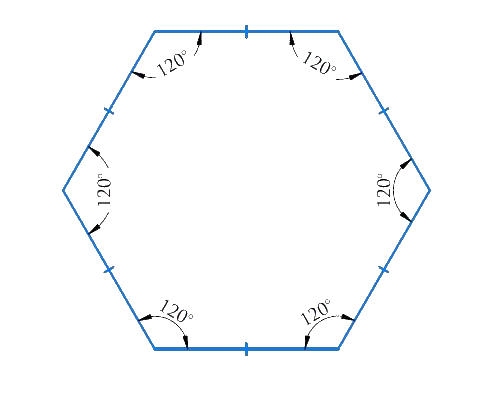## Chapter 3 Ex.3.1 Question 6

Find the angle measure $$x$$ in the following figures: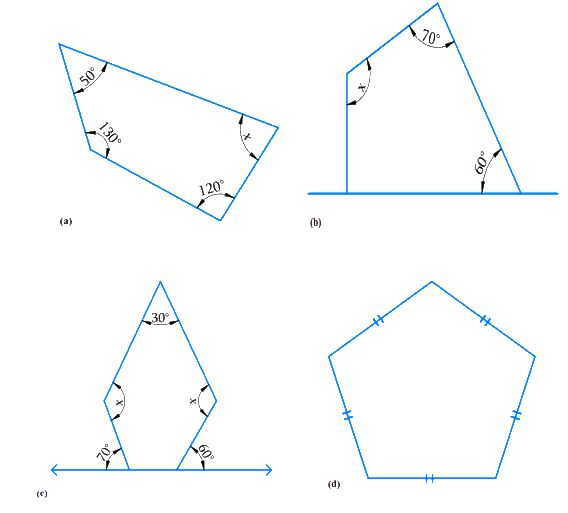### Solution

What is Known?

Sum of the measures of all interior angles of a quadrilateral is $$360^\circ$$ and that of a pentagon is $$540^\circ$$

What is Unknown?

Angle $$x$$ in the above figures  $$a, b, c$$ and $$d$$

Steps: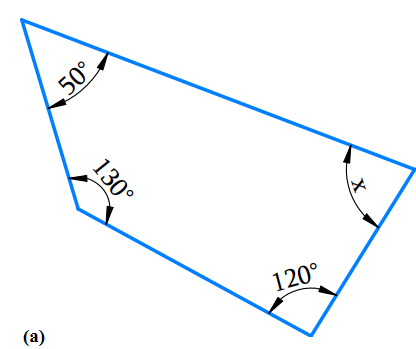a) The above figure has $$4$$ sides and hence it is a quadrilateral.

Using the angle sum property of a quadrilateral,

\begin{align}{{50}^{\rm{o}}} + {{130}^{\rm{o}}} + {{120}^{\rm{o}}} + x &= {{360}^{\rm{o}}}\\ {{300}^{\rm{o}}} + x &= {{360}^{\rm{o}}}\\x & = {{360}^{\rm{o}}} - {{300}^{\rm{o}}}\\x &= {{60}^{\rm{o}}} \end{align}

b) Using the angle sum property of a quadrilateral.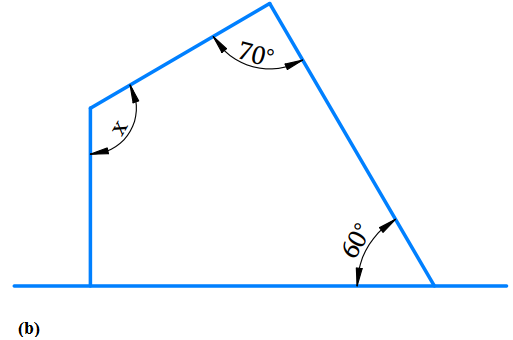\begin{align}{{90}^\circ } + {{60}^\circ } + {{70}^\circ } + x& = {{360}^\circ }\\{{220}^\circ } + x &= {{360}^\circ }\\{220^\circ } + x &= {360^\circ }\\x& = {360^\circ } - {220^\circ }\\x &= {140^\circ }\end{align}

c) The given figure is a pentagon $$(n=5)$$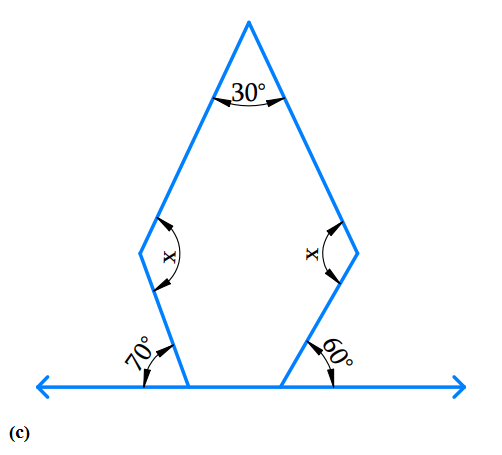Angle sum of a polygon

\begin{align}& = ({\rm{n} - 2) \times {{180}^{\rm{o}}}}\\&=(5 - 2) \times {{180}^{\rm{o}}}\\& =3 \times {{180}^{\rm{o}}}\\ & = {540}^{\rm{o}}\end{align}

Sum of the interior angle of pentagon is $$540^\circ$$

Angles at the bottom are linear pair.

$$\therefore$$ First base interior angle a

\begin{align}& = {180^{\rm{o}}} - {70^{\rm{o}}}{\rm{ }}\left( \begin{array}{l}{\text{angle of straight }}\\{\text{line is }}{180^{\rm{o}}}\end{array} \right)\\ &= {\mkern 1mu} {\mkern 1mu} {110^{\rm{o}}} \end{align}

$$\therefore$$  Second base interior angle b

\begin{align}&= {{180}^{\rm{o}} - {{60}^{\rm{o}}}}\\& = {{120}^{\rm{o}}}\end{align}

Angle sum of a polygon $$= ({\rm{n}} - 2) \times {180^o}$$

\begin{align}\\{30^o} + x + {110^o} + {120^o} + x &= {540^o}\\2x + {260^{\rm{o}}}\, &= {540^{\rm{o}}}\\2x &\!=\!{540^{\rm{o}}}\!-\!{260^{\rm{o}}}\\2x &= {280^{\rm{o}}}\\x &= \frac{{{{280}^{\rm{o}}}}}{2}\\x &= {140^{\rm{o}}}\end{align}

D) The given figure is pentagon $$(n-5)$$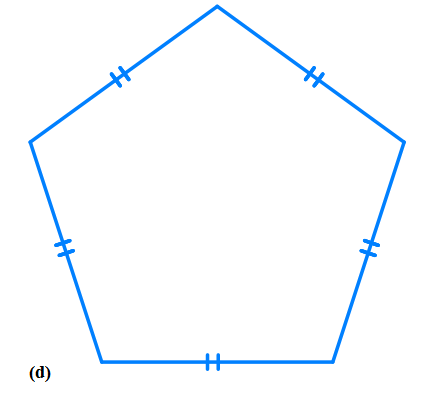Angle sum of a polygon

\begin{align}& = ({\rm{n}} - 2) \times {{180}^{\rm{o}}}\\& = (5 - 2) \times {{180}^{\rm{o}}}\\& = 3 \times {{180}^{\rm{o}}}\\& = {{540}^{\rm{o}}}\end{align}

Sum of the interior angle of pentagon is $$540^\circ$$

Angle sum of a polygon

\begin{align}&= {x + x + x + x + x = {{540}^{\rm{o}}}}\\{\rm{ i}}{\rm{.e}}{\rm{. }}\ \qquad 5x &= {{540}^{\rm{o}}}\\x &= {\frac{{{{540}^{\rm{o}}}}}{5}}\\x & = {{108}^{\rm{o}}}\end{align}

A pentagon is a regular polygon i.e. it is both ‘equilateral’ and ‘equiangular’. Thus, the measure of each interior angle of the pentagon are equal.

Hence each interior angle is $$108^\circ .$$

## Chapter 3 Ex.3.1 Question 7

a) Find $$x +y +z$$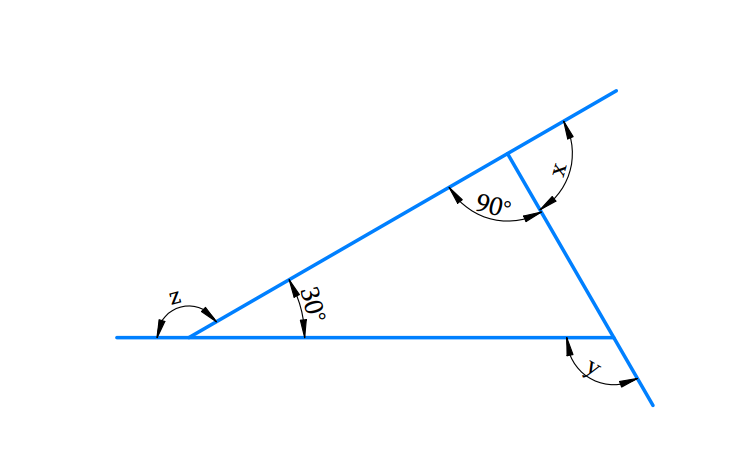b) Find $$x +y +z +w$$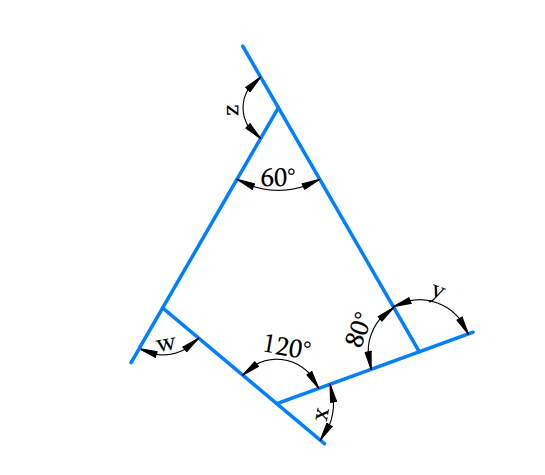### Solution

What is Known?

The sum of the measures of all the interior angles of a quadrilateral is $$360^\circ$$ and that of a triangle is $$180^\circ$$

What is Unknown?

Angle$$x, y, z$$ and $$w$$ in the above figures $$a$$ and $$b$$

Reasoning:

The unknown angles can be estimated by using the angle sum property of a quadrilateral and triangle accordingly.

Steps:

(a) Find $$x +y +z$$Sum of linear pair of angles is$$= {180^{\rm{o}}}$$

\begin{align}x + {90^{\rm{o}}} & = {180^{\rm{o}}}{\text{ (Linear pair)}}\\x & = {180^{\rm{o}}} - {90^{\rm{o}}}\\x & = {90^{\rm{o}}}\end{align}

And

\begin{align}z + 30^{\rm{o}} &= {180^{\rm{o}}}{\rm{ }}({\text{Linear pair}})\\z & = 180^{\rm{o}} - {30^{\rm{o}}}\\z &= {150^{\rm{o}}}\end{align}

And

\begin{align}y & ={{90}^{\text{o}}}+{{30}^{\text{o}}}\text{ }\left( \begin{array}&\text{Exterior angle } \\ \text{theorem} \\ \end{array} \right) \\ y & ={{120}^{\text{o}}} \\\end{align}

\begin{align}x + y + z & = {90^{\rm{o}}} + {120^{\rm{o}}} + {150^{\rm{o}}}\\& = {360^{\rm{o}}}\end{align}

(b)Find $$x +y +z$$The sum of the measures of all the interior angles of a quadrilateral is $$360^\circ.$$

Using the angle sum property of a quadrilateral,

Let $$n$$ is the fourth interior angle of the quadrilateral.

\begin{align}6{{0}^{\text{o}}}+8{{0}^{o}}+12{{0}^{o}}+n &=36{{0}^{o}} \\26{{0}^{o}}+n& =36{{0}^{o}} \\n& =36{{0}^{o}}-26{{0}^{o}} \\n& =10{{0}^{o}} \\\end{align}

sum of linear pair of angles is $$= {180^{\rm{o}}}$$

\begin{align}w + 100^\circ & = 180^\circ {\rm{ }} & \ldots \left( 1 \right)\\x + 120^\circ & = 180^\circ & \ldots \left( 2 \right)\\y + 80^\circ & = 180^\circ & \ldots \left( 3 \right)\\z + 60^\circ & = 180^\circ & \ldots \left( 4 \right)\end{align}

Adding equation ($$1), (2), (3)$$and $$(4),$$

\begin{align} & \left( \begin{array} & w\!+\!{{100}^{{}^\circ }}\!+\!x+{{120}^{{}^\circ }} \\ \!+\!y\!+\!{{80}^{{}^\circ }}\!+\!z+{{60}^{{}^\circ }} \\ \end{array} \right)\!\!=\!\!\left( \begin{array} & {{180}^{{}^\circ }}\!+\!{{180}^{{}^\circ }}\!+\! \\ {{180}^{{}^\circ }}\!+\!{{180}^{{}^\circ }} \\ \end{array} \right) \\&\,\, \quad w+x+y+z+{{360}^{{}^\circ }}={{720}^{{}^\circ }} \\ &\,\,\qquad \qquad w+x+y+z={{720}^{{}^\circ }}-{{360}^{{}^\circ }} \\ & \qquad \quad\,\,\; w+x+y+z={{360}^{{}^\circ }} \end{align}

The sum of the measures of the external angles of any polygon is $$360^\circ$$ .